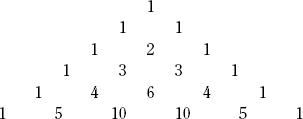NextPrevious

# How is Pascal’s triangle used?

Pascal’s triangle is an array of numbers, arranged so that every number is equal to the sum of the two numbers above it on either side. It can be represented in several slightly different triangles, but this is the most common form:

The triangle is used to determine the numerical coefficients resulting from the computation of higher powers of a binomial (two numbers added together). When a binomial is raised to a higher power, the result is expanded, using the numbers in that row of the triangle. For example, (a b)1 = a1 b1, using the coefficients in the second line of the triangle (a b)2 = a2 2ab b2, using the coefficients in the next line of the triangle. (The first line of the triangle correlates to [a b]0.) While the calculation of coefficients is fairly straightforward, the triangle is useful in calculating them for the higher powers without needing to multiply them out. Binomial coefficients are useful in calculating probabilities; Blaise Pascal was one of the pioneers in developing laws of probability.

As with many other mathematical developments, there is some evidence of a previous appearance of the triangle in China. Around 1100C.E., the Chinese mathematician Chia Hsien wrote about “the tabulation system for unlocking binomial coefficients”; the first publication of the triangle was probably in a book called Piling-Up Powers and Unlocking Coefficients by Liu Ju-Hsieh.Close

This is a web preview of the "The Handy Science Answer Book" app. Many features only work on your mobile device. If you like what you see, we hope you will consider buying. Get the App